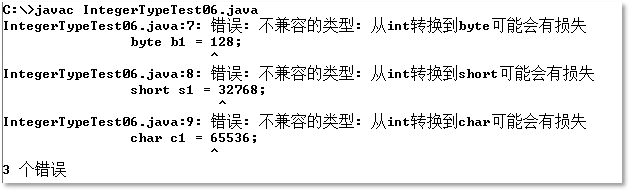java整数型详解

1. 十进制：0,1,2,3,4,5,6,7,8,9,10,11,12,13,14,15,16,17...
2. 八进制：0,1,2,3,4,5,6,7,10,11,12,13,14,15,16,17,20,21...
3. 十六进制：0,1,2,3,4,5,6,7,8,9,a,b,c,d,e,f,10,11...

public class IntegerTypeTest01 {
public static void main(String[] args) {
//十进制
int a = 10;
System.out.println("a = " + a);
//八进制
int b = 010;
System.out.println("b = " + b);
//十六进制
int c = 0x10;
System.out.println("c = " + c);
}
}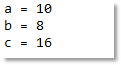public class IntegerTypeTest02 {
public static void main(String[] args) {

//声明一个int类型的变量a
//100被默认当做int类型处理
//以下代码不存在类型转换
int a = 100;

//100被默认当做int类型处理，占用4个字节
//b变量是long类型，默认可容纳8个字节
//小容量转换成大容量，叫做自动类型转换
long b = 100;

//b变量long类型，占用8个字节
//c变量int类型，占用4个字节
//大容量不能直接赋值给小容量，编译报错了
//int c = b;

//强制类型转换需要添加强制类型转换符
//强制类型转换时可能引起精度损失，谨慎使用
int c = (int)b;

//a是int类型
//b是long类型
//c是int类型
//多种数据类型混合运算时先转换成容量最大的再做运算
//最终的结果是long类型，大容量无法直接赋值给小容量
//所以编译报错了
//int d = a + b + c;

//解决以上编译错误
int d = (int)(a + b + c);
System.out.println("d = " + d);
//或者
long d2 = a + b + c;
System.out.println("d2 = " + d2);

//100L被当做long类型处理
//x变量是long类型
//不存在类型转换
long x = 100L;
System.out.println("x = " + x);
}
}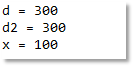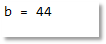4个字节的int类型300强转为1个字节的byte类型，最终的结果是44，为什么呢？首先300对应的二进制码是：00000000 00000000 00000001 00101100，强制类型转换的时候会变成1个字节，这个时候底层是将前3个字节砍掉了，也就是最后的二进制码是：00101100，这个二进制码对应的是44。

public class IntegerTypeTest03 {
public static void main(String[] args) {
int a = 300;
byte b = (byte)a;
System.out.println("b = " + b);
}
}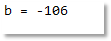public class IntegerTypeTest05 {
public static void main(String[] args) {
long num = 2147483648;
}
}public class IntegerTypeTest06 {
public static void main(String[] args) {
byte b = 127;
short s = 32767;
char c = 65535;

byte b1 = 128;
short s1 = 32768;
char c1 = 65536;
}
}